Home
Hostname: page-component-55b6f6c457-pc5cw Total loading time: 0.225 Render date: 2021-09-27T17:24:49.772Z Has data issue: true Feature Flags: { "shouldUseShareProductTool": true, "shouldUseHypothesis": true, "isUnsiloEnabled": true, "metricsAbstractViews": false, "figures": true, "newCiteModal": false, "newCitedByModal": true, "newEcommerce": true, "newUsageEvents": true }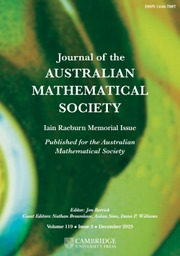Journal of the Australian Mathematical Society

# AN ARITHMETIC EQUIVALENCE OF THE RIEMANN HYPOTHESIS

Published online by Cambridge University Press:  18 June 2018

## Abstract

Let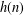$h(n)$ denote the largest product of distinct primes whose sum does not exceed$n$. The main result of this paper is that the property for all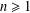$n\geq 1$, we have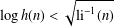$\log h(n)<\sqrt{\text{li}^{-1}(n)}$ (where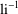$\text{li}^{-1}$ denotes the inverse function of the logarithmic integral) is equivalent to the Riemann hypothesis.

## MSC classification

Type
Research Article
Information
© 2018 Australian Mathematical Publishing Association Inc.

## Access options

Get access to the full version of this content by using one of the access options below. (Log in options will check for institutional or personal access. Content may require purchase if you do not have access.)

## Footnotes

Research partially supported by CNRS, Institut Camille Jordan, UMR 5208.

## References

Abramowitz, M. and Stegun, I. A., Handbook of Mathematical Functions (Dover Publications, New York).CrossRefGoogle Scholar
Axler, C., ‘New bounds for the sum of the first$n$ prime numbers’, J. Théor. Nombres Bordeaux (to appear) (2016), arXiv:1606.06874.Google Scholar
Axler, C., ‘New estimates for some functions defined over primes’, Intergers (to appear) (2017),arXiv:1703.08032.Google Scholar
Berndt, B. C., Ramanujan’s Notebooks, Part IV (Springer, New York, 1994), 126131.CrossRefGoogle Scholar
Cohen, H., Number Theory, Analytic and Modern Tools, Volume II (Springer, New York, 2007).Google Scholar
Deléglise, M. and Nicolas, J.-L., ‘Le plus grand facteur premier de la fonction de Landau’, Ramanujan J. 27 (2012), 109145.CrossRefGoogle Scholar
Deléglise, M. and Nicolas, J.-L., ‘Maximal product of primes whose sum is bounded’, Proc. Steklov Inst. Math. 282 (2013), 73102; (Issue 1, Supplement).CrossRefGoogle Scholar
Deléglise, M. and Nicolas, J.-L., ‘On the largest product of primes with bounded sums’, J. Integer Sequences 18 (2015), Article 15.2.8.Google Scholar
Deléglise, M., Nicolas, J.-L. and Zimmermann, P., ‘Landau’s function for one million billions’, J. Théor. Nombres Bordeaux 20 (2008), 625671.CrossRefGoogle Scholar
Deléglise, M. and Rivat, J., ‘Computing 𝜋(x): The Meissel, Lehmer, Lagarias, Miller, Odlyzko method’, Math. Comp. 65 (1996), 235245.CrossRefGoogle Scholar
Deléglise, M. and Rivat, J., ‘Computing 𝜓(x)’, Math. Comp. 67 (1998), 16911696.CrossRefGoogle Scholar
Dusart, P., ‘Explicit estimates of some functions over primes’, Ramanujan J. 45 (2018), 227251.CrossRefGoogle Scholar
Edwards, H. M., Riemann’s Zeta Function (Academic Press, New York, 1974).Google Scholar
Ellison, W. J. and Ellison, F., Prime Numbers (Wiley, New York, 1985).Google Scholar
Ellison, W. J. and Mendès France, M., Les nombres premiers (Hermann, Paris, 1975).Google Scholar
Landau, E., Handbuch der Lehre von der Verteilung der Primzahlen, I, 2nd edn (Chelsea, New York, 1953).Google Scholar
Massias, J.-P. and Robin, G., ‘Bornes effectives pour certaines fonctions concernant les nombres premiers’, J. Théor. Nombres Bordeaux 8 (1996), 215242.CrossRefGoogle Scholar
Massias, J.-P., Nicolas, J.-L. and Robin, G., ‘Évaluation asymptotique de l’ordre maximum d’un élément du groupe symétrique’, Acta Arith. 50 (1988), 221242.CrossRefGoogle Scholar
Massias, J.-P., Nicolas, J.-L. and Robin, G., ‘Effective bounds for the maximal order of an element in the symmetric group’, Math. Comp. 53(188) (1989), 665678.CrossRefGoogle Scholar
Nicolas, J.-L., ‘Small values of the Euler function and the Riemann hypothesis’, Acta Arith. 155 (2012), 311321.CrossRefGoogle Scholar
Platt, D. J. and Trudgian, T., ‘On the first sign change of 𝜃(x) - x ’, Math. Comp. 85(299) (2016), 15391547.CrossRefGoogle Scholar
Robin, G., ‘Grandes valeurs de la fonction somme des diviseurs et hypothèse de Riemann’, J. Math. Pures Appl. 63 (1984), 187213.Google Scholar
Salvy, B., ‘Fast computation of some asymptotic functional inverses’, J. Symbolic Comput. 17 (1994), 227236.CrossRefGoogle Scholar
Schoenfeld, L., ‘Sharper bounds for the Chebyshev functions 𝜃(x) and 𝜓(x) II’, Math. Comp. 30 (1976), 337360.Google Scholar

# Send article to Kindle

Note you can select to send to either the @free.kindle.com or @kindle.com variations. ‘@free.kindle.com’ emails are free but can only be sent to your device when it is connected to wi-fi. ‘@kindle.com’ emails can be delivered even when you are not connected to wi-fi, but note that service fees apply.

Find out more about the Kindle Personal Document Service.

AN ARITHMETIC EQUIVALENCE OF THE RIEMANN HYPOTHESIS
Available formats
×

# Send article to Dropbox

To send this article to your Dropbox account, please select one or more formats and confirm that you agree to abide by our usage policies. If this is the first time you use this feature, you will be asked to authorise Cambridge Core to connect with your <service> account. Find out more about sending content to Dropbox.

AN ARITHMETIC EQUIVALENCE OF THE RIEMANN HYPOTHESIS
Available formats
×

# Send article to Google Drive

To send this article to your Google Drive account, please select one or more formats and confirm that you agree to abide by our usage policies. If this is the first time you use this feature, you will be asked to authorise Cambridge Core to connect with your <service> account. Find out more about sending content to Google Drive.

AN ARITHMETIC EQUIVALENCE OF THE RIEMANN HYPOTHESIS
Available formats
×
×